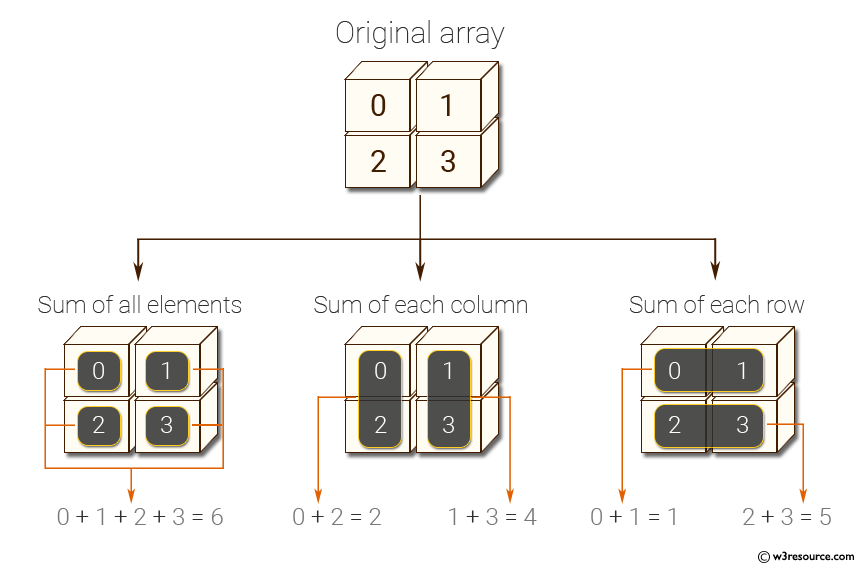﻿ NumPy: Compute sum of all elements, sum of each column and sum of each row of a given array - w3resource# NumPy: Compute sum of all elements, sum of each column and sum of each row of a given array

## NumPy: Basic Exercise-32 with Solution

Write a NumPy program to compute sum of all elements, sum of each column and sum of each row of a given array.

Sample Solution :

Python Code :

``````import numpy as np
x = np.array([[0,1],[2,3]])
print("Original array:")
print(x)
print("Sum of all elements:")
print(np.sum(x))
print("Sum of each column:")
print(np.sum(x, axis=0))
print("Sum of each row:")
print(np.sum(x, axis=1))
``````

Sample Output:

```Original array:
[[0 1]
[2 3]]
Sum of all elements:
6
Sum of each column:
[2 4]
Sum of each row:
[1 5]
```

Pictorial Presentation:Python Code Editor:

Have another way to solve this solution? Contribute your code (and comments) through Disqus.

What is the difficulty level of this exercise?

Test your Python skills with w3resource's quiz

﻿

## Python: Tips of the Day

Returns the symmetric difference between two lists, after applying the provided function to each list element of both

Example:

```def tips_symmetric_difference_by(p, q, fn):
_p, _q = set(map(fn, p)), set(map(fn, q))
return [item for item in p if fn(item) not in _q] + [item for item in q if fn(item) not in _p]
from math import floor
print(tips_symmetric_difference_by([4.2, 2.4], [4.6, 6.8],floor))
```

Output:

```[2.4, 6.8]
```